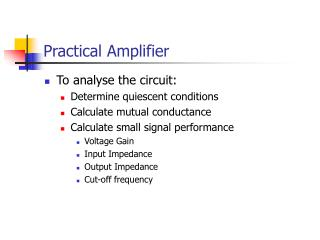DownloadDownload PresentationPractical Amplifier

# Practical Amplifier

Télécharger la présentation## Practical Amplifier

- - - - - - - - - - - - - - - - - - - - - - - - - - - E N D - - - - - - - - - - - - - - - - - - - - - - - - - - -
##### Presentation Transcript

1. Practical Amplifier • To analyse the circuit: • Determine quiescent conditions • Calculate mutual conductance • Calculate small signal performance • Voltage Gain • Input Impedance • Output Impedance • Cut-off frequency

2. Quiescent Conditions

3. Small Signal Analysis: Voltage Gain As before:

4. Input and Output Impedance • Unlike the op-amp, transistor amplifiers have significant output impedances and finite input impedances • RIN can be comparable with the source resistance of the input signal • ROUT can be comparable with the load resistance

5. Input Impedance iIN iB • Input impedance, rIN, is the ratio of the small signal input voltage and the small signal input current iRB

6. Input Impedance (cont) iIN iB iRB

7. Output Impedance • One way to measure rOUT is: • Short the input to 0 V • Output now looks like just rOUT

8. Applying Kirchoff’s current law: By Ohm’s law: Output Impedance (cont)

9. Coupling Capacitors • Capacitor COUT is needed to remove the d.c. component of the collector voltage • Capacitor CIN is needed to allow the base voltage to be offset from 0V • In both cases this is known as coupling • Both capacitors are chosen to look like short circuits at operating frequencies • Their reactance will, however, become significant at low frequencies

10. Equivalent Circuit

11. If the cut-off frequency, fC, is specified and rIN has been calculated: Cut-Off Frequency Cut-off frequency, or –3dB point, is when the gain of the amplifier falls by a factor of Ö2 NB. This assumes that COUT still looks like a short circuit

12. Typically, choose: COUT • For the lower cut-off frequency calculation to be valid, COUT should still look like a short circuit at fC

13. But, where, Also, Emitter Capacitor For the highest voltage gain, vBE vIN vE

14. To make sure, choose, Emitter Capacitor (cont) For CE to not interfere at fC: vBR Where, vIN vE NB. Use rE (=VT/IC) not RE for this calculation!

15. Summary • In the context of the common-emitter amplifier we have covered: • Small signal analysis • Mutual conductance • Input/output impedance • Coupling capacitor requirements and cut-off frequencies • Next time: • Applying the same principles to the differential amplifier • It’s actually a much easier circuit to analyse – honest! • Make sure you’re happy with the fundamentals by then!